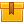# Introduction to AC Components and Motors

None.

## Description

This is the first lesson in the iKNOW® AC/DC Motor Theory Library. This lesson identifies the components of an AC motor and explains their functions. Basic magnetic principles, sine waves, methods of increasing magnetic flux in a conductor, and how a rotating field is created in an AC Motor are presented.

## Objectives

Identify the components of an AC Motor and explain their function. Explain the basic principles of magnetism. Interpret the characteristics of a current as represented on a sine wave. Describe the effect of AC current on a conductor. Describe the methods of increasing magnetic flux in a conductor. Explain how a rotating field is created in an AC Motor.

## ContentThis is the first lesson in the iKNOW™ AC/DC Motor Theory Library. This
lesson identifies the components of an AC motor and explains their
functions. Basic magnetic principles, sine waves, methods of increasing
magnetic flux in a conductor, and how a rotating field is created in an
AC Motor are presented.

•Introduction to AC Components and Motors

0
0 Reviews